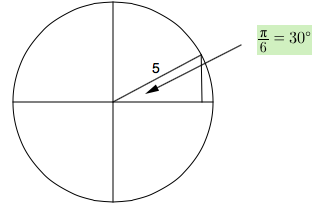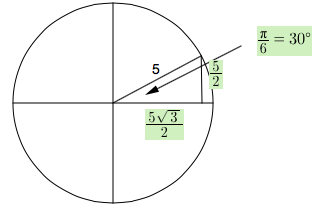### Home > PC > Chapter 10 > Lesson 10.1.3 > Problem10-39

10-39.

Find r in component form if $θ$ is the standard angle.

1. $\| \textbf{r} \|=5$ and $θ = \frac { \pi } { 6 }$

Draw each vector in a circle. Then make a right triangle with the $x$-axis.

Circle, radius in first quadrant, labeled 5, is hypotenuse in right triangle where horizontal leg is part of x axis, central angle of triangle labeled 1 sixth pi = 30 degrees.

Solve the rest of the triangle.
Note: Each part forms a special right triangle.

Labels added, horizontal leg, 5 halves times square root of 3, vertical leg, 5 halves.2. $\| \textbf{r} \|=10$ and $θ = \frac { 5 \pi } { 4 }$

3. $\| \textbf{r} \|=15$ and $θ = \frac { 2 \pi } { 3 }$